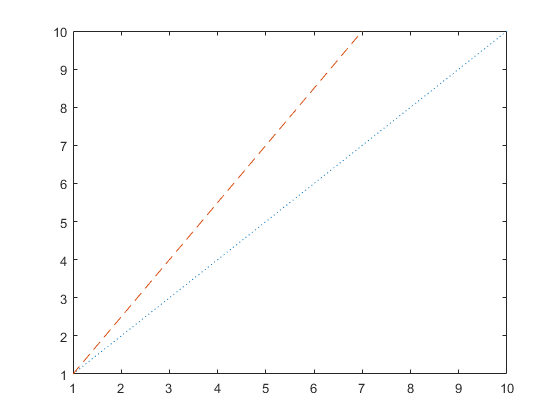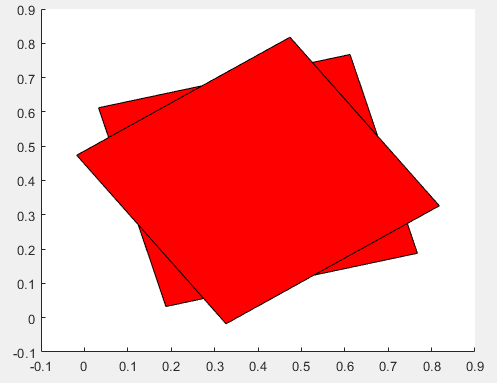# arguments

Declare function argument validation

## Syntax

```arguments
`argName1 (dimensions) class {validators} = defaultValue`
...
`argNameN ...`
end

arguments (Repeating)
`argName1 (dimensions) class {validators} = defaultValue`
...
`argNameN ...`
end

arguments (Output)
`argName1 (dimensions) class {validators}`
...
`argNameN ...`
end

arguments (Output,Repeating)
`argName (dimensions) class {validators}`
end

```

## Description

### Input Argument Blocks

example

````arguments ... end` declares input arguments for a function. The arguments block is optional. If you include one or more `arguments` blocks, they must appear before the first executable line of the function. MATLAB® treats any argument block that is not labeled explicitly with `Input` or `Output` as an input block.Each argument can have one or more restrictions or a default value, as shown in this syntax:```argName (dimensions) class {validators} = defaultValue``` `(dimensions)` — Input size, specified as a comma-separated list of two or more numbers, such as `(1,2)`, `(3,5,2)`, or `(1,:)`. A colon allows any length in that dimension. `(dimensions)` cannot include expressions.The dimensions of the input must match `(dimensions)` exactly or be compatible with the size specified by `(dimensions)`. For example, `(1,:)` specifies the input must be a 1-by-n row vector, but an n-by-1 column vector is compatible. The function reshapes a row vector input into a column vector. Similarly, a size of `(2,3)` allows scalar input, but it expands the input to a 2-by-3 matrix. See Compatible Array Sizes for Basic Operations for more information.`class` — Class or MATLAB data type specified by name, such as `double`. The input must be the specified type or a type that can be converted to that type. For example, a function that specifies `double` accepts values of class `single` and converts them to `double`. For more information on conversions, see Implicit Class Conversion.`{validators}` — Comma-separated list of validation functions, such as `mustBeNumeric` and `mustBeScalarOrEmpty`, enclosed in curly brackets. Validation functions error when the input arguments do not match their conditions. Unlike `class`, validation functions do not modify input arguments. For a list of validation functions, see Argument Validation Functions.`defaultValue` — Default values must conform to the specified size, type, and validation rules. A default value can also be an expression. Specifying a default value makes the argument optional. Optional arguments must be positioned after required arguments in the function signature and in the `arguments` block. For name-value arguments, `arg` uses the form `nv.name`, where `nv` is a structure name in the function signature and `name` is the argument name in the arguments block. For instance, define a function that accepts name-value arguments using a structure named `options`. y = myFunction(x,options) In the arguments block, specify the names for name-value arguments as fields:arguments x options.Name1 options.Name2 end For more information on using `arguments` blocks in general, see arguments Block Syntax.```

example

````arguments (Repeating) ... end` declares repeating input arguments.For example, if you create a function named `myplot` with repeating arguments `X`, `Y`, and `style`, the function accepts multiple sets of these three arguments, such as `myplot(x1,y1,style1,x2,y2,style2)`. MATLAB creates a cell array that contains all the values passed in for that argument. Functions can include only one repeating input arguments block. If the function includes both repeating and name-value arguments, declare name-value arguments in their own, separate arguments block after the repeating arguments block.For more information on repeating arguments, see Repeating Arguments.```

### Output Argument Blocks

example

````arguments (Output) ... end` declares output arguments for a function. The output arguments block is optional. If you include one or more output `arguments` blocks, they must appear after all input blocks but before the first executable line of the function. When including both input an output blocks in a function, including the `(Input)` and `(Output)` attributes explicitly is recommended for readability. See Repeating Outputs with Argument Validation for an example.(since R2022b)Like input arguments, output arguments can have one or more restrictions, as shown in this syntax:```argName (dimensions) class {validators}```See the description for `arguments ... end` for additional details. Unlike input arguments, output arguments cannot define a default value, and validation functions applied to an output argument cannot reference an earlier output argument.```

example

``` `arguments (Output,Repeating) ... end` declares a repeating output argument for a function. You can use argument validation for a repeating output argument, but you can define only one repeating output argument per function. `varargout` can appear in a repeating output arguments block as long as it is the only output argument. (since R2022b)```

## Examples

collapse all

Write a function that restricts the size of the input argument to a row vector of any length. Use a validation function to restrict the elements of that vector to numeric values.

```function [m,s] = twoStats(x) arguments x (1,:) {mustBeNumeric} end m = mean(x,"all"); s = std(x,1,"all"); end```

Call the function on a three-element row vector.

```a = [1 3 5]; [m,s] = twoStats(a)```
```m = 3 s = 1.6330```

Calling the function with a column vector is also valid because row and column vectors are compatible.

```a = [1 3 5]'; [m,s] = twoStats(a)```
```m = 3 s = 1.6330```

If you call the function with a vector that contains nonnumeric values, the `mustBeNumeric` validation function throws an error.

```a = ["1" "3" "5"]; [m,s] = twoStats(a)```
```Error using twoStats Invalid argument at position 1. Value must be numeric.```

To declare optional name-value arguments for a function, include a structure name in the function declaration, and define the argument names as fields of that structure in the `arguments` block.

Declare the `myRectangle` function with `options` as a structure name. The two fields of `options`, `LineStyle` and `LineWidth`, are the names in the function’s name-value arguments:

```function myRectangle(X,Y,options) arguments X double Y double options.LineStyle (1,1) string = "-" options.LineWidth (1,1) {mustBeNumeric} = 1 end % Function code ... end ```

Both of the argument names have defined default values, so they are both optional. All of these syntaxes are valid ways to call the function:

```myRectangle(4,5) myRectangle(4,5,LineStyle=":",LineWidth=2) myRectangle(4,5,LineWidth=2,LineStyle=":") myRectangle(4,5,LineStyle=":") myRectangle(4,5,LineWidth=2) ```

Before R2021a, pass names as strings or character vectors, and separate names and values with commas. Both syntaxes are valid in later releases.

Repeating arguments are single arguments or groups of arguments that can be repeated zero or more times in a function call. The `fRepeat` function accepts repeating groups of arguments `x`, `y`, and `style`. Restrict the input arguments `x` and `y` to vectors of double values or values convertible to doubles. Restrict `style` to the strings `"--"` and `":"` .

```function fRepeat(x,y,style) arguments (Repeating) x (1,:) double y (1,:) double style {mustBeMember(style,["--",":"])} end % Reshape the cell arrays of inputs and call plot function z = reshape([x;y;style],1,[]); if ~isempty(z) plot(z{:}); end end```

Call `fRepeat` with two groups of inputs. MATLAB creates a cell array containing all the values passed in for `x`, another array for the values of `y`, and a third for the values of `style`. The function then reshapes those arrays into a 1-by-6 cell array, `z`, and passes it to `plot`.

```x1 = 1:10; y1 = 1:10; s1 = ":"; x2 = 1:7; y2 = 1:1.5:10; s2 = "--"; fRepeat(x1,y1,s1,x2,y2,s2) ```Write a function that rotates a two-dimensional square patch about point (0.4, 0.4) by a user-specified number of degrees. Return x- and y-coordinates of the final image as output arguments, and restrict those values to be positive. In other words, the function should return coordinates only when the final result of the rotation is entirely in the first quadrant.

```function [xfinal,yfinal] = rotatePatch(angle) arguments (Output) xfinal {mustBePositive} yfinal {mustBePositive} end x = [0.1 0.1 0.7 0.7]; y = [0.1 0.7 0.7 0.1]; p = patch(x,y,"red"); rotate(p,[0 0 1],angle,[0.4 0.4 0]) xfinal = p.Vertices(:,1); yfinal = p.Vertices(:,2); end```

Call the function with an angle of 15 degrees. This rotation does not move any of the vertices out of the first quadrant, so the function returns without error.

`[x1,y1] = rotatePatch(15)`
```x1 = 0.1879 0.0326 0.6121 0.7674 y1 = 0.0326 0.6121 0.7674 0.1879```Call the function with an angle of 35 degrees, which moves the lower-left vertex out of the first quadrant. The negative x-coordinate does not satisfy the output argument validation, so the function returns an error.

`[x2,y2] = rotatePatch(35)`
```Invalid output 'xfinal'. Value must be positive. Error in rotatePatch (line 12) end```Write a function that accepts repeating pairs of vectors and returns the sum of each pair. Restrict the inputs and outputs to row vectors.

```function vectorSum = repeatSum(a,b) arguments (Input,Repeating) a (1,:) b (1,:) end arguments (Output,Repeating) vectorSum (1,:) end n = numel(a); vectorSum{n} = a{n} + b{n}; for i = 1:n-1 vectorSum{i} = a{i} + b{i}; end end ```

Calculating the final output and assigning it to `vectorSum{n}` before the `for`-loop preallocates space for the cell array. Expanding the cell array in the loop without preallocation can have a negative effect on performance.

Define two pairs of vectors. Call `repeatSum` on with the two pairs as input. The input arguments block validation converts column vectors to row vectors because they compatible sizes.

```x1 = [1 2]; y1 = [3 4]; x2 = [1; 0]; y2 = [0; 1]; [sum1,sum2] = repeatSum(x1,y1,x2,y2)```
```sum1 = 4 6 sum2 = 1 1```

Because the inputs are restricted to row vectors, the sum of each pair of vectors is always a row vector. However, the output validation helps ensure that the function produces row vectors even if the function is revised at a later date. For example, if the input validation were changed to the `mustBeVector` function, pairs could be composed of one row vector and one column vector without conversion. In that case, the sum of `x1` and `y2` is a matrix.

`x1 + y2`
```ans = 1 2 2 3 ```

The output of the revised `repeatSum` would error because the matrix would not pass the output validation.

## Limitations

• Argument blocks are not supported in nested functions, abstract methods, or handle class destructor methods.

collapse all

### Supported Data Types

Argument declarations can specify any MATLAB class or externally defined class that is supported by MATLAB, except Java classes, COM classes, and MATLAB classes defined before MATLAB software Version 7.6 (in other words, class definitions that do not use the `classdef` keyword).

## Tips

• Using data type restrictions can result in implicit conversions of input arguments. For example:

```function y = myFunction(inputArg1) arguments inputArg1 (1,1) double end ...```
For this function, if you pass the string `"123"` as the input argument, MATLAB converts the string to the numeric value `123` of type `double`.

Validation functions do not change input values in any way, so to avoid data type conversion, use one or more validator functions instead of a data type to restrict the input. For example:

• To avoid conversion of strings to numeric values, use `mustBeA`, `mustBeFloat`, or `mustBeNumeric`.

• To avoid conversion of numeric values to strings, use `mustBeText`, `mustBeTextScalar`, or `mustBeNonZeroLengthText`.

• To avoid size conversions, use `mustBeVector` or `mustBeScalarOrEmpty`.

## Version History

Introduced in R2019b

expand all